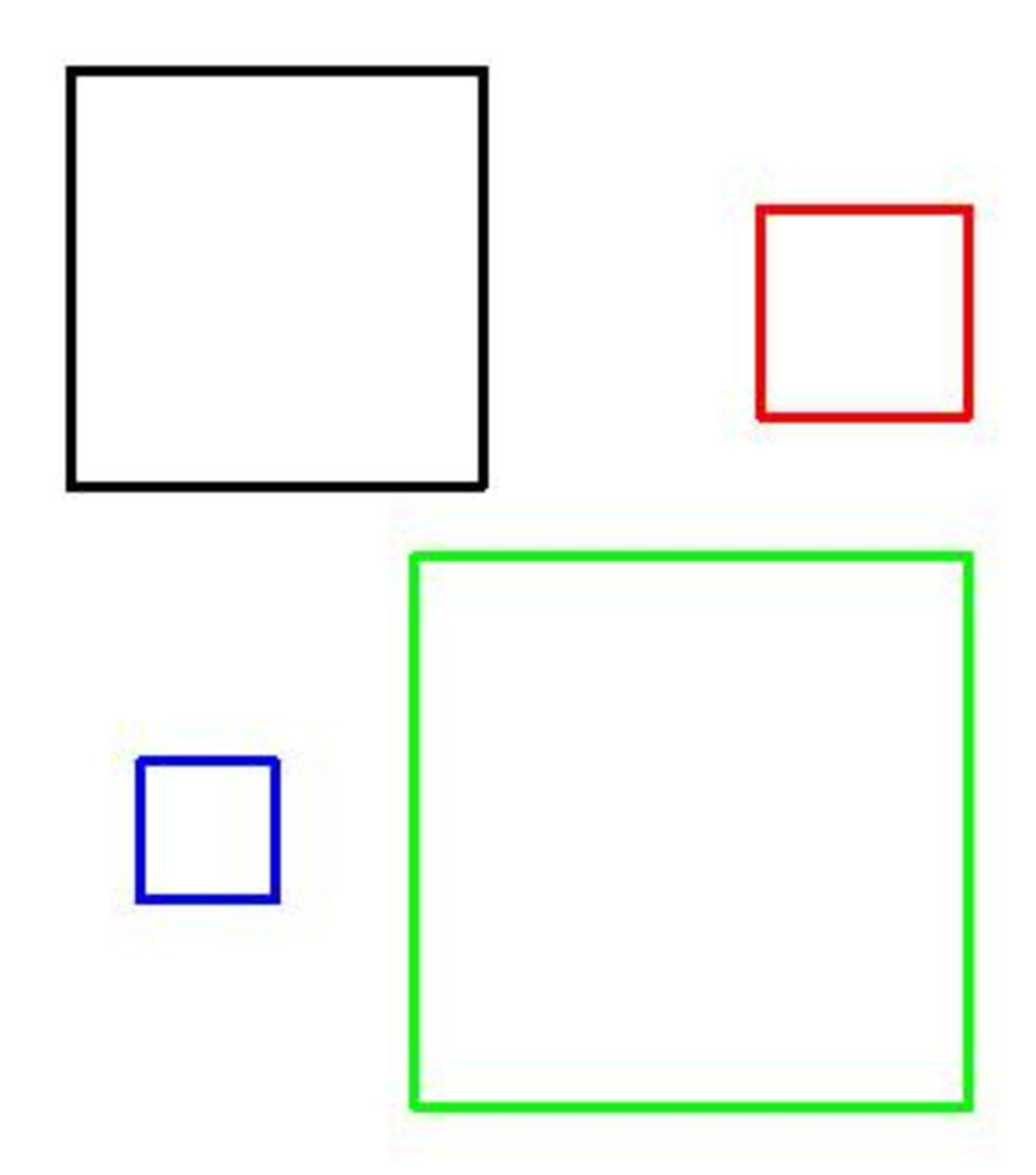Updated date:

# What are the math facts about a square? (mathematical properties of a square)

Author:A square is probably one of the first mathematical shapes that you will recognise. Here are a few more mathematical facts about a square:

A square has four equal side lengths.

All the interior angles of a square are 90⁰ (right angles).

Since all the sides and angles are the same size, a square can also be referred to as a regular quadrilateral.

A square also has 4 lines of reflective symmetry; one vertical, one horizontal and two diagonal lines of symmetry.

A square also has rotational symmetry. The order of rotational symmetry of a square is 4. This means it rotates onto itself 4 times in a full turn.

To calculate the perimeter of square all you need to do is multiply one of the side lengths by 4. So if you call one of the sides x then the perimeter of the square will be 4x. For example, a square of side length 7 cm will have a perimeter of 28 cm (since 4 × 7 = 28)

The area of the square can be found by squaring one of the side lengths. So if you call one of the sides x, then the area will be x². For example, a square of side length 8cm will have an area of 64cm². There are also 10,000 cm² contained in a 1m² (since 100 × 100 = 10,000).

The diagonals of a square (a line drawn from one vertex to another) meet at the centre of the shape and make four right angles at their point of intersection.

A square is one of the easiest shapes to tessellate as you can fit 4 squares around a single point (since 360⁰ ÷ 90⁰ = 4). You will find tessellations of squares on kitchen floors, bathroom floors, patios and driveways around the world.

## Comments

Helen Murphy Howell from Fife, Scotland on November 17, 2011:

Very interesting hub. Many thanks for sharing!

rlaha from Spartanburg, SC on November 16, 2011:

Useful and informative! Thanks!

William Norman from Cross Plains, Texas on November 16, 2011:

To find the length of a diagonal on a square, multiply the side length by the square root of 2.

Joan Whetzel on November 16, 2011:

As usual, another great Hub with easy to understand information.